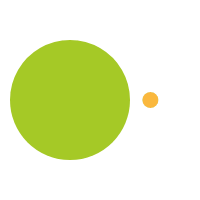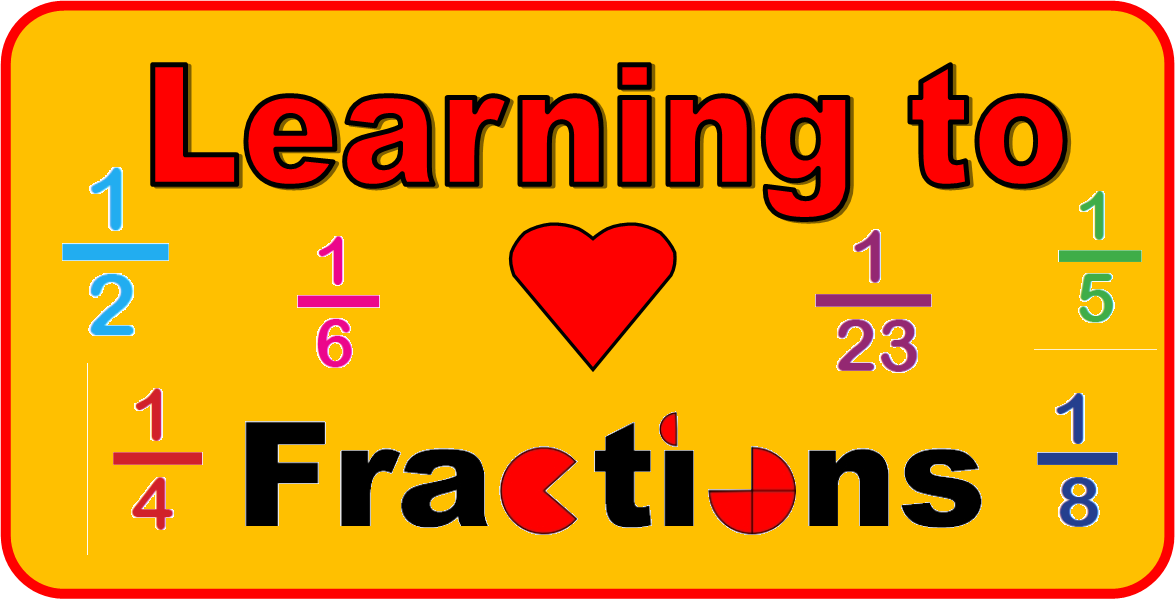February 7, 2019

# Fractions!

There’s a fine line between a numerator and a denominator in a fraction and 4 out of 3 people won’t get it.

Most teachers will agree, students don’t like fractions, BUT…  they are the most crucial aspect of students’ mathematical growth.  In working with educators, they lament about student’s resistance to fractions, the trouble students have making sense of them and applying them to problem solving.

“The losses that occur because of the gaps in conceptual understanding about fractions, ratios, and related topics are incalculable.  The consequences of doing, rather than understanding, directly or indirectly affect a person’s attitudes towards mathematics, enjoyment and motivation in learning, course selection in mathematics and science, achievement, career flexibility and even the ability to fully appreciate some of the simplest phenomena in everyday life.” Susan J. Lamon (2012, p.xi)

## There are three fundamental reasons we must take a closer look at how we teach fractions in the United States.

1. Fractions play a key role in students’ feelings about mathematics. For many students this is the first time they struggle with math and applying what they have memorized in a different format.
2. Fractions are fundamental to school math and daily life. Fractions underpin many complex topics including ratios, rates, percents, proportions, proportionality, linearity and slope.  They are also incorporated in many daily life activities; following a recipe, calculating discount, splitting a bill, comparing rates, converting measuring units, investing money, using tools and applying them to household tasks.
3. Fractions are foundational to success in algebra. In its final report, Foundations for Success, the National Mathematics Advisory Panel (2008) concluded that (1) algebra is the gateway to success in high school and college, and (2) the main reason for U.S. students’ failure in algebra is their poor proficiency with fractions. And STEM careers are unattainable without passing algebra.

# Let’s talk numbers!

As an elementary teacher you might be saying, aren’t rational numbers a middle school concept?  Elementary students work with whole numbers not rational number.   “Rational numbers compose a major area of school mathematics that is crucial for students to learn but challenging for teachers to teach.  Students in grades 3 – 5 need to understand rational numbers well if they are to succeed in these grades and in their subsequent mathematics experiences” (Barnett-Clarke, Fisher, Marks, & Ross, 2010,p.1).

Understanding numbers in the primary grades helps to rationalize, rational numbers.  In kindergarten through second grade students work in the additive world where they are combining objects or decomposing numbers.  If we add 1 to each number to get the next number, we can hold and count and touch the quantities.  Student then move in 3rd grade to measuring such things as length, area and time, quantities where whole numbers are broken down into rational numbers.   But the challenge is to create an environment where students stop applying what they know about whole numbers to rational numbers.

Example:               1/4 is greater than 1/3, because 4 is greater than 3

2/3 + 1/2 = 3/5, because 2 + 1 =3 and 3 + 2 + 5

2/3 x 9 can’t be 6 because multiplying makes numbers bigger

4 ÷ 1/2 can’t be 8 because division makes numbers smaller.

So often with learning about working with rational numbers students are quickly rushed to algorithms told to “just follow these steps: find common denominators then add/subtract”, “ours is not to reason why, just invert and multiply”, “when dividing, cross multiply”.  This leads to frustration, surrender and even failure.  The human mind is curious and seeks to know why but so often in mathematics and working with rational numbers teachers often go straight to the algorithm.  There is no time to work on the whys, we have to much material to cover. Plus, many of us were never taught the whys.

# So how can we help students succeed at fractions?

First let’s introduce them to what a fraction is early.  Food is a great place to start.  Do you want half a sandwich?  Can you measure 3/4 a cup of milk?  If you eat ¼ of this pizza, how much is left?  All this helps to set the idea that a fraction is a part of a “whole” something.

There is a game called Izzi, by Thinkfun, which has puzzle pieces with squares that are partially black and white.  I set all the pieces out and ask the students to categorize each tile as either ½ black, less than ½ black or greater than ½ black.

The puzzle pieces look like this

I like this activity because it involves students thinking, communicating and collaborating.  Is the first tile greater than, less than or equal to 1/2?  How do you know? What about the second tile? Third tile?

Pattern Blocks are also a great way to help students understand parts of a whole and equivalent fractions.  Along with the standard pattern blocks, which can represent 1/2, 1/3, 1/6 and 1 whole, you can purchase additional pieces that represent 1/4 and 1/12.  Students can play with these pieces and discuss how many pieces make a whole (yellow hexagon).  To expand on this, students can replace pieces with other shapes that cover the same (equivalent) space.  Three green triangles cover the same space as one red trapezoid, one blue rhombus covers the same space as two green triangles.

Creating a game with the pattern block fraction pieces has the students practicing the skills of fraction recognition and equivalent fractions.  Here is an example of such a game, Cookie Munch.  Students think of the pattern blocks as cookie pieces and take part of each cookie away based on a roll of the dice.  The students enjoy the game and practice equivalent fractions.

Another frequently difficult concept for students to understand is that was addressed above is, “1/4 is greater than 1/3, because 4 is greater than 3”.  This is a frequent misconception that number means size and not quantity, “4 is larger than 3”, “what is one larger than 3”.  Having students playing with fractional pieces to recognize that the “larger” the denominator the more pieces there are of the whole, 1/12 is one of twelve pieces, then comparing that to 1/2 of the same whole.

“Same whole”, that is another concept that can be addressed at a young age.  Is ½ of a cake the same as ½ of a cupcake? Showing wholes that are different sizes is necessary.  Grade school students see fractions only in similar imagery like,

They need to see fractions beyond pizza, circles and rectangles.

Linear measurement can be introduced as early as third grade with students using rulers to measure to the nearest ¼ of an inch.

Students love searching for hidden treasure.  This activity that has students drawing line segments on a map in the search of treasure is a great way for them to recognize mixed numbers. Measure a line segment that is 5 ¾ inches long has students recognizing that they need 5 whole inches and then ¾ of an inch.  For students to solve for total segments measured they can use visual representations of pattern blocks or Legos to determine the total amount measured as they can create the whole numbers and then add on the fractional numbers using specific pattern blocks that represent ¼, ½, ¾.

One of the third-grade common core standards is solve problems involving measurement and estimation of intervals of time and liquid volumes. A great way to introduce relevant fractions to students is with the Gallon Man and with a clock.

The gallon man can be made easily with 5 sheets of construction paper.  First whole piece is the body and represents 1 gallon (this also can be done with actual gallon milk jugs, ½ gallon jug, quart carton, pint carton and a small cup carton).  The second sheet of paper is the ½ gallon and the student fold a paper in half and create the head.  Next paper students fold it in half and then half again, cut on the lines and they have 1/4th or quarts.  Next sheet of paper the students will fold in half, then half again and then one more time for 8 squares or 1/8ths, cutting on the line and pints on all of them.  The final piece of paper is folded in half four times.  When unfolded there will be 16 squares that need to be cut out and cups written on them.  A cup is 1/16 of a gallon.  The cups can be compared to the pints and quarts to see how many cover the space to determine equivalencies.

Time can also be broken down into fractional amounts.  And students can use it for telling time and understanding why we say “quarter past”, “half past”.

If you are interested in math activities and games like these, check out our website www.mangomath.com and make sure to follow us on social media for the latest and greatest math information.